# General

The aim of the package `plot.matrix` is to visualize a matrix as is with a heatmap. Automatic reordering of rows and columns is only done if necessary. This is different as in similar function like `heatmap`. Additionally it should be user-friendly and give access to a lot of options if necessary.

Currently the package implements the S3 functions below such that you can use the generic `plot` function to plot matrices as heatmaps:

• `plot.matrix` for a heatmap for a plain matrix,
• `plot.loadings` for a heatmap for a loadings matrix from factor analysis or principal component analysis (reordering of rows!).

The plot itself is composed by a heatmap (usually left) where colors represent matrix entries and a key (usually right) which links the colors to the values.

## First examples

``````library('plot.matrix')
# numeric matrix
x <- matrix(runif(35), ncol=5) # create a numeric matrix object
class(x)
#>  "matrix" "array"
par(mar=c(5.1, 4.1, 4.1, 4.1)) # adapt margins
plot(x)``````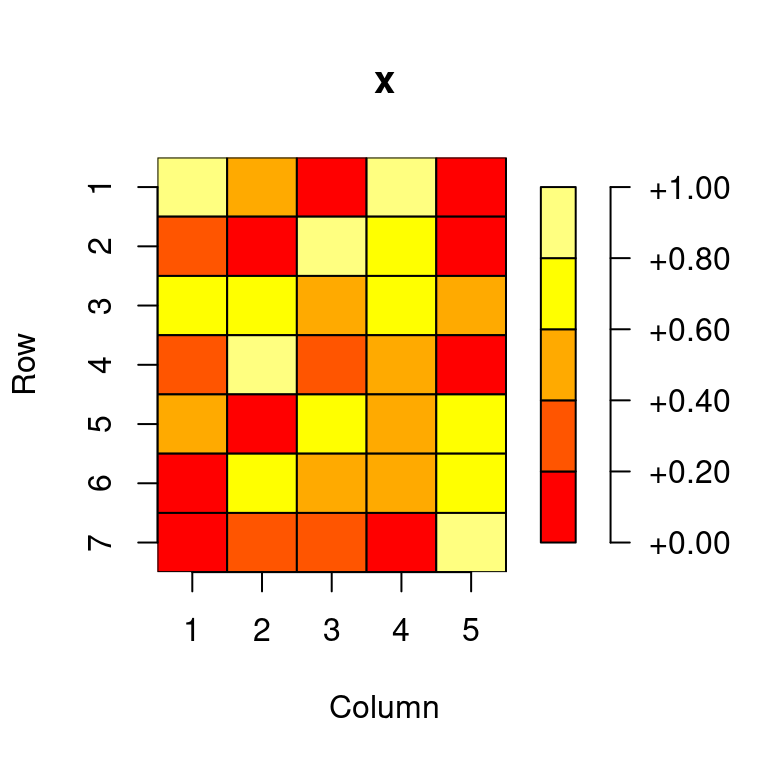``````# logical matrix
m <- matrix(runif(35)<0.5, ncol=7)
plot(m)``````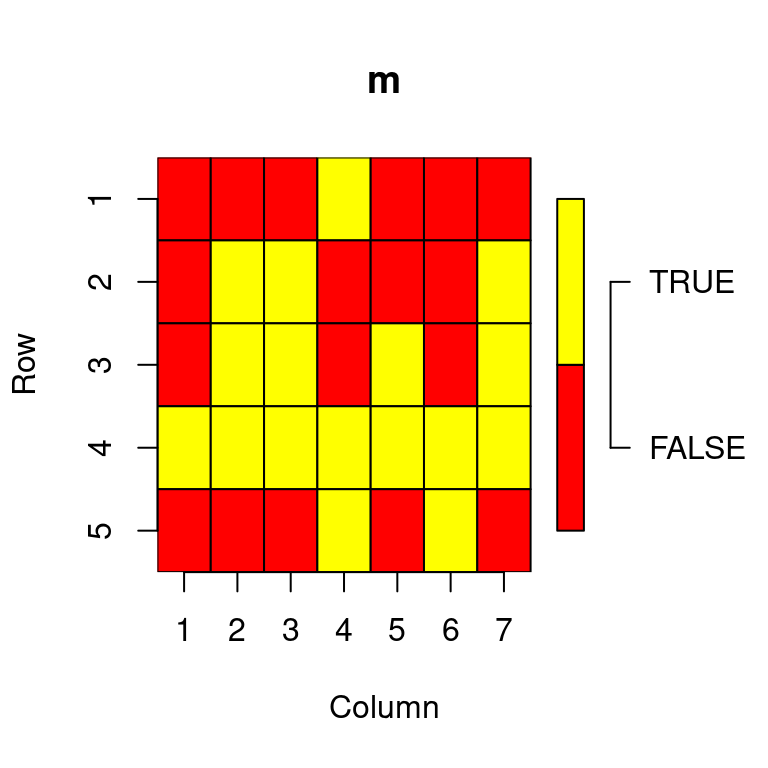``````# text matrix
s <- matrix(sample(letters[1:10], 35, replace=TRUE), ncol=5)
plot(s)``````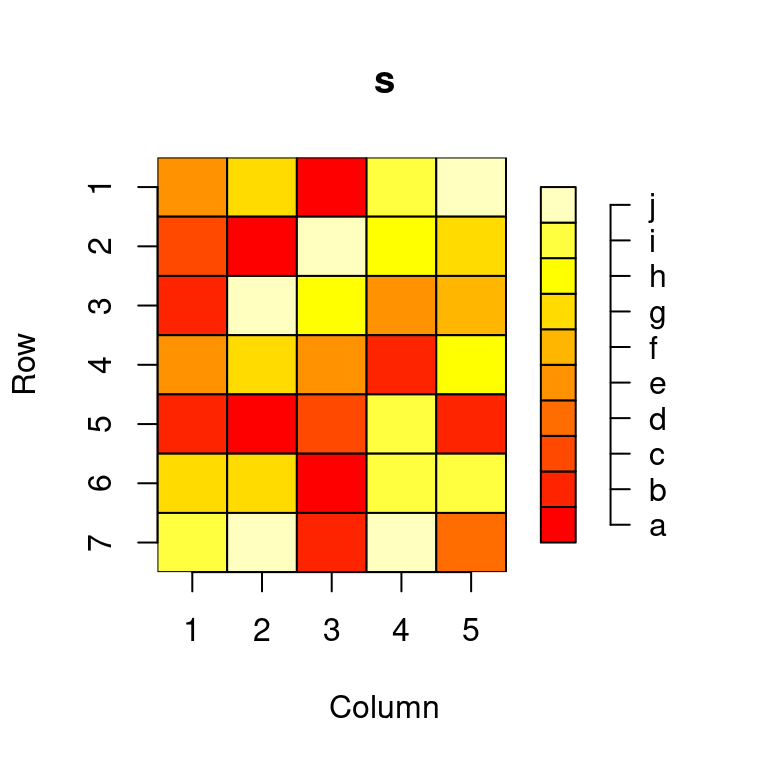``````library('plot.matrix')
library('psych')
data <- na.omit(bfi[,1:25])
fa <- fa(data, 5, rotate="varimax")
par(mar=c(5.1, 4.1, 4.1, 4.1)) # adapt margins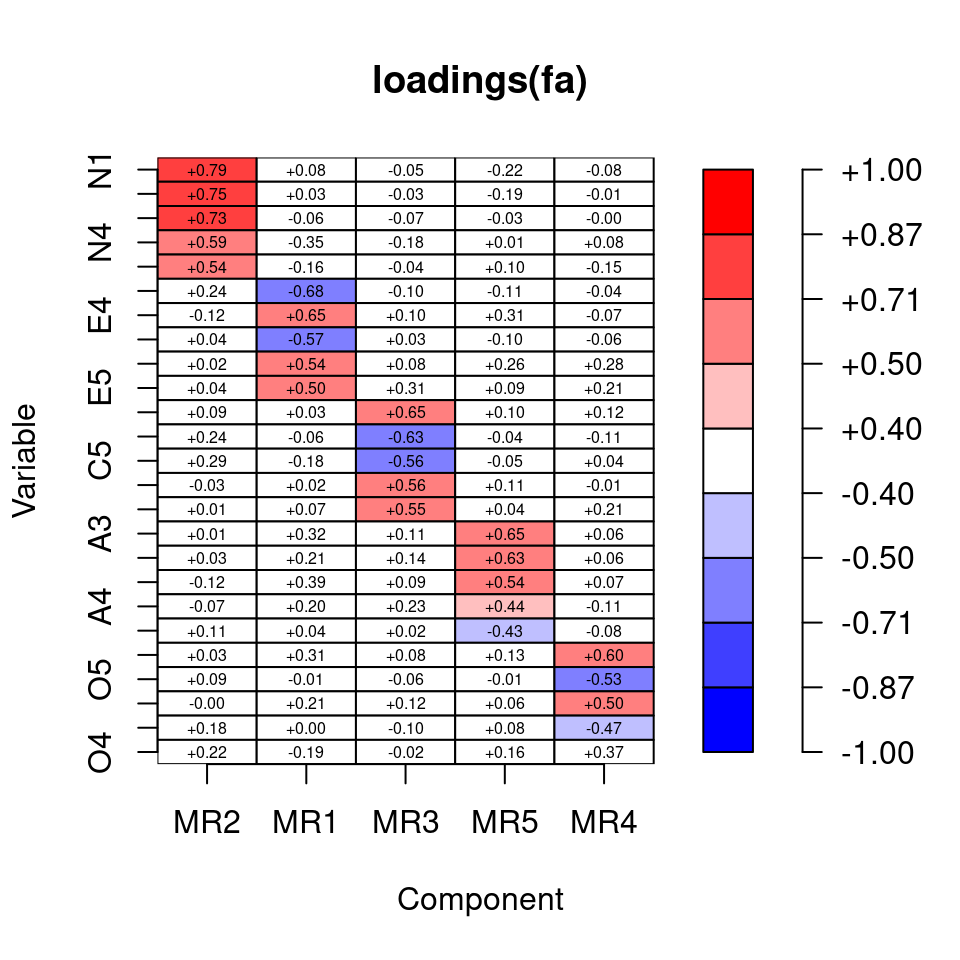## Assigning colors and breaks

`plot.matrix` uses the command `assignColors`, also part of `plot.matrix`, assigns to each value in `x` a color based on the parameters `breaks`, `col` and `na.col` given.

In case of a numeric matrix `breaks` can be

• a number, giving the number of intervals covering the range of `x`,
• a vector of two numbers, given the range to cover with 10 intervals, or
• a vector with more than two numbers, specify the interval borders

In case of a non-numeric vector `breaks` must contain all values which are will get a color. If `breaks` is not given then a sensible default is chosen: in case of a numeric vector derived from `pretty` and otherwise all unique values/levels are used.

`col` can be either be a vector of colors or a function which generates via `col(n)` a set of `n` colors. The default is to use `heat.colors`.

## Choosing color palettes/functions

In case that you want to provide your own color palettes/functions for plotting there are several good choices within R packages: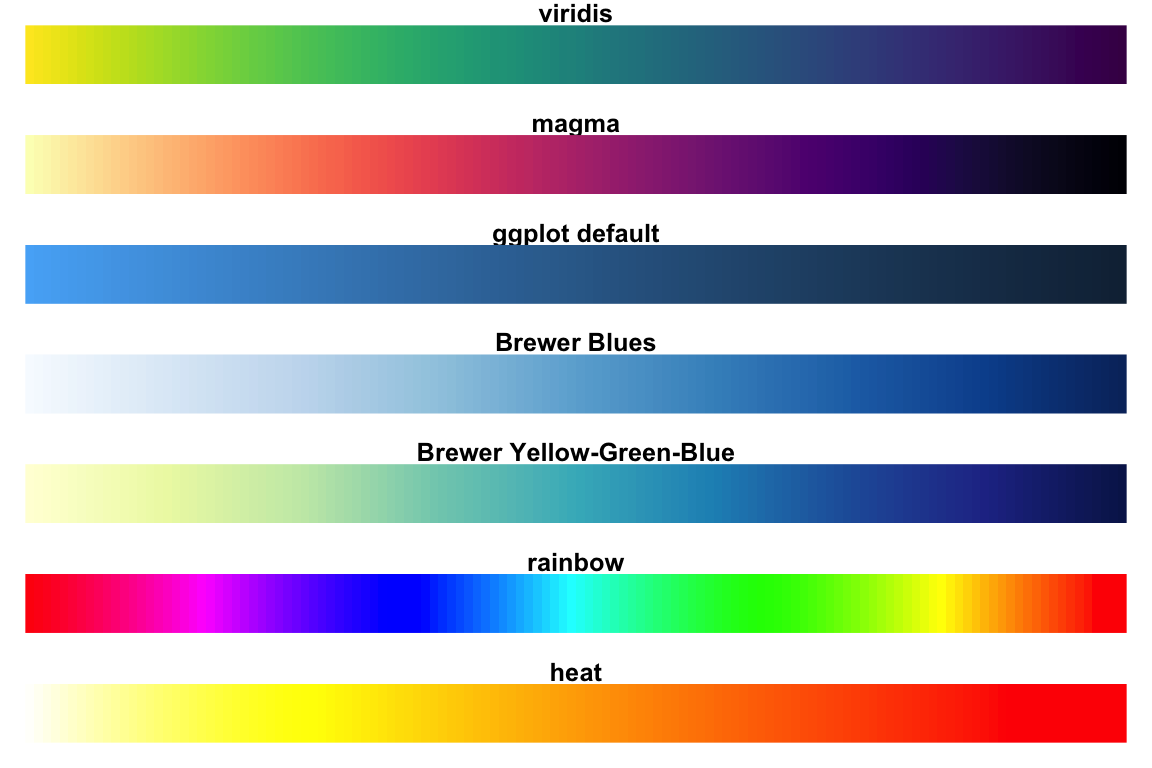## Structure of the plot

The plot is created in several steps

1. a call to the `plot` command to create the basic plot
2. draw colored polygons for each matrix entry with the `polygon` command
3. if necessary add the value of each matrix entry with the `text` command in a polygon
4. if necessary draw x- and y-axis with the `axis` command into the plot
5. if necessary draw the key with the `axis` and the `polygon` command

## Formal parameters

 plot.matrix( x, y = NULL, breaks = NULL, col = heat.colors, na.col = “white”, na.cell = TRUE, na.print = TRUE, digits = NA, fmt.cell = NULL, fmt.key = NULL, spacing.key = c(1, 0.5, 0), polygon.cell = NULL, polygon.key = NULL, text.cell = NULL, key = list(side = 4, las = 1), axis.col = list(side = 1), axis.row = list(side = 2), axis.key = NULL, max.col = 70, …)

You may influence the appearance by setting your own parameters:

1. `...` all parameters given here will be given to the `plot` command, e.g. `xlab`, `ylab`, ….
2. `polygon.cell` list of parameters for drawing polygons for matrix entries
3. `text.cell` list of parameters for putting for matrix entries as texts
4. `axis.col` and `axis.row` list of parameters for drawing for row and column axes
5. `key`, `axis.key`, `spacing.key` and `polygon.key` to draw the key
6. `max.col` to determine when text color and background color to near

## Set global parameters

You may set global parameters for all subsequent calls of `axis`, `polygon` and `text` via the `...`. The following parameters are supported

function parameter(s)
`axis` `cex.axis`, `col.axis`, `col.ticks`, `font`, `font.axis`, `hadj`, `las`, `lwd.ticks`, `line` , `outer`, `padj`, `tck`, `tcl`, `tick`
`polygon` `angle`, `border`, `density`
`text` `cex`, `font`, `vfont`
``````par(mar=c(5.1, 4.1, 4.1, 4.1)) # adapt margins
# omit all borders
plot(x, border=NA)``````